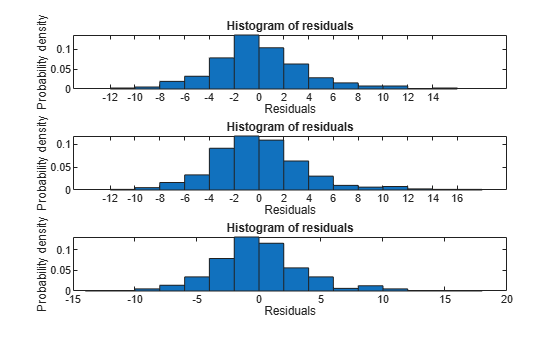## Stepwise Regression

### Stepwise Regression to Select Appropriate Models

`stepwiselm` creates a linear model and automatically adds to or trims the model. To create a small model, start from a constant model. To create a large model, start with a model containing many terms. A large model usually has lower error as measured by the fit to the original data, but might not have any advantage in predicting new data.

`stepwiselm` can use all the name-value options from `fitlm`, with additional options relating to the starting and bounding models. In particular:

• For a small model, start with the default lower bounding model: `'constant'` (a model that has no predictor terms).

• The default upper bounding model has linear terms and interaction terms (products of pairs of predictors). For an upper bounding model that also includes squared terms, set the `Upper` name-value pair to `'quadratic'`.

### Compare large and small stepwise models

This example shows how to compare models that `stepwiselm` returns starting from a constant model and starting from a full interaction model.

Load the `carbig` data and create a table from some of the data.

```load carbig tbl = table(Acceleration,Displacement,Horsepower,Weight,MPG);```

Create a mileage model stepwise starting from the constant model.

`mdl1 = stepwiselm(tbl,'constant','ResponseVar','MPG')`
```1. Adding Weight, FStat = 888.8507, pValue = 2.9728e-103 2. Adding Horsepower, FStat = 3.8217, pValue = 0.00049608 3. Adding Horsepower:Weight, FStat = 64.8709, pValue = 9.93362e-15 ```
```mdl1 = Linear regression model: MPG ~ 1 + Horsepower*Weight Estimated Coefficients: Estimate SE tStat pValue __________ __________ _______ __________ (Intercept) 63.558 2.3429 27.127 1.2343e-91 Horsepower -0.25084 0.027279 -9.1952 2.3226e-18 Weight -0.010772 0.00077381 -13.921 5.1372e-36 Horsepower:Weight 5.3554e-05 6.6491e-06 8.0542 9.9336e-15 Number of observations: 392, Error degrees of freedom: 388 Root Mean Squared Error: 3.93 R-squared: 0.748, Adjusted R-Squared: 0.746 F-statistic vs. constant model: 385, p-value = 7.26e-116 ```

Create a mileage model stepwise starting from the full interaction model.

`mdl2 = stepwiselm(tbl,'interactions','ResponseVar','MPG')`
```1. Removing Acceleration:Displacement, FStat = 0.024186, pValue = 0.8765 2. Removing Displacement:Weight, FStat = 0.33103, pValue = 0.56539 3. Removing Acceleration:Horsepower, FStat = 1.7334, pValue = 0.18876 4. Removing Acceleration:Weight, FStat = 0.93269, pValue = 0.33477 5. Removing Horsepower:Weight, FStat = 0.64486, pValue = 0.42245 ```
```mdl2 = Linear regression model: MPG ~ 1 + Acceleration + Weight + Displacement*Horsepower Estimated Coefficients: Estimate SE tStat pValue __________ __________ _______ __________ (Intercept) 61.285 2.8052 21.847 1.8593e-69 Acceleration -0.34401 0.11862 -2.9 0.0039445 Displacement -0.081198 0.010071 -8.0623 9.5014e-15 Horsepower -0.24313 0.026068 -9.3265 8.6556e-19 Weight -0.0014367 0.00084041 -1.7095 0.088166 Displacement:Horsepower 0.00054236 5.7987e-05 9.3531 7.0527e-19 Number of observations: 392, Error degrees of freedom: 386 Root Mean Squared Error: 3.84 R-squared: 0.761, Adjusted R-Squared: 0.758 F-statistic vs. constant model: 246, p-value = 1.32e-117 ```

Notice that:

• `mdl1` has four coefficients (the `Estimate` column), and `mdl2` has six coefficients.

• The adjusted R-squared of `mdl1` is `0.746`, which is slightly less (worse) than that of `mdl2`, `0.758`.

Create a mileage model stepwise with a full quadratic model as the upper bound, starting from the full quadratic model:

`mdl3 = stepwiselm(tbl,'quadratic','ResponseVar','MPG','Upper','quadratic');`
```1. Removing Acceleration:Horsepower, FStat = 0.075209, pValue = 0.78405 2. Removing Acceleration:Weight, FStat = 0.072756, pValue = 0.78751 3. Removing Horsepower:Weight, FStat = 0.12569, pValue = 0.72314 4. Removing Weight^2, FStat = 1.194, pValue = 0.27521 5. Removing Displacement:Weight, FStat = 1.2839, pValue = 0.25789 6. Removing Displacement^2, FStat = 2.069, pValue = 0.15114 7. Removing Horsepower^2, FStat = 0.74063, pValue = 0.39 ```

Compare the three model complexities by examining their formulas.

`mdl1.Formula`
```ans = MPG ~ 1 + Horsepower*Weight ```
`mdl2.Formula`
```ans = MPG ~ 1 + Acceleration + Weight + Displacement*Horsepower ```
`mdl3.Formula`
```ans = MPG ~ 1 + Weight + Acceleration*Displacement + Displacement*Horsepower + Acceleration^2 ```

The adjusted ${R}^{2}$ values improve slightly as the models become more complex:

```RSquared = [mdl1.Rsquared.Adjusted, ... mdl2.Rsquared.Adjusted, mdl3.Rsquared.Adjusted]```
```RSquared = 1×3 0.7465 0.7580 0.7599 ```

Compare residual plots of the three models.

```subplot(3,1,1) plotResiduals(mdl1) subplot(3,1,2) plotResiduals(mdl2) subplot(3,1,3) plotResiduals(mdl3)```The models have similar residuals. It is not clear which fits the data better.

Interestingly, the more complex models have larger maximum deviations of the residuals:

```Rrange1 = [min(mdl1.Residuals.Raw),max(mdl1.Residuals.Raw)]; Rrange2 = [min(mdl2.Residuals.Raw),max(mdl2.Residuals.Raw)]; Rrange3 = [min(mdl3.Residuals.Raw),max(mdl3.Residuals.Raw)]; Rranges = [Rrange1;Rrange2;Rrange3]```
```Rranges = 3×2 -10.7725 14.7314 -11.4407 16.7562 -12.2723 16.7927 ```